## 2009年5月27日 星期三

### good::gx介面

good::gx是負責繪圖的介面。它很簡單，至少目前來說還很簡單。只有二個介面：Image及Graphics。

Image介面對貼圖作一層薄薄的包裝，good執行的時候，所有使用到的貼圖的地方全都透過這一層介面作存取。下面是Image介面定義。
`template<class ImgT>class Image{public:  bool isValid() const;  int getWidth() const;  int getHeight() const;  bool hasKeyColor() const;  int getKeyColor() const;  void setKeyColor(int keycolor);};`
Graphics介面提供了很少的功能，目前只有貼圖和畫色塊二個功能，因為目前good的成像目前只需要這二個功能就足夠畫出所有東西。下面是Graphics介面定義。
`template<class ImgT>class Graphics{public:  bool drawImage(int x, int y, ImgT const& img, int srcx, int srcy, int srcw, int srch);  bool drawImage(int x, int y, ImgT const& img)  {      return drawImage(x, y, img, 0, 0, img.getWidth(), img.getHeight());  }  bool fillSolidColor(int left, int top, int width, int height, int color);};`
+ + +

## 2009年5月22日 星期五

### OpenGL 畫色塊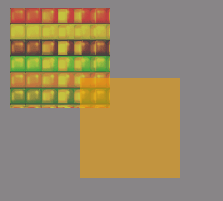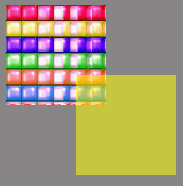+ + +

## 2009年5月17日 星期日

### 編輯魔法寶石遊戲資源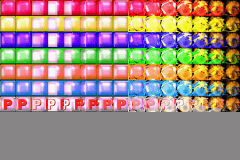+ + +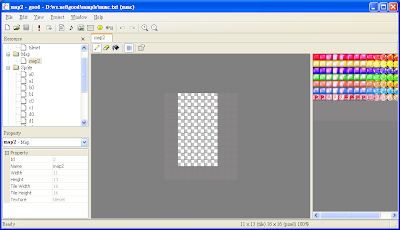## 2009年5月13日 星期三

### 快速複製STL Stream內容

`ifstream ins("in.txt");ofstream outs("out.txt");outs << ins.rdbuf();`

## 2009年5月10日 星期日

### 魔法寶石消除演算法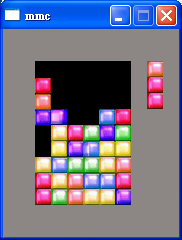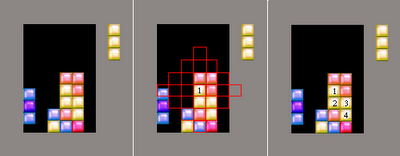## 2009年5月6日 星期三

### good的資料格式

good專案資料格式使用Ini，再透過smallworld2的Ini模組就能很簡單的操作讀寫。

+ + +

`[good]version=0.3name=mmcwindow=176 208texs=1maps=2 3sprites=4 5 6 7 8 9 10 11 12 13 14 15levels=18`

Script資源

`[scripts]16=./mmc.lua`

`[tex5]name=tilesetfileName=./weeder.bmphasKeyColor=1keyColor=253 0 255`

`[map26]width=6height=6texture=5tileWidth=32tileHeight=32cxTile=8cyTile=8data=k2VgYJBEw1JALA/ElmhYgQhxayLVy2CxVxqIAQ==vgrid=1 128 128 128hgrid=1 128 128 128`

data欄位看起來是個亂它八糟的字串，這是編碼過的地圖資料。每張地圖是width乘height個Tile所組成，假如地圖比較大的話那要把所有資料都列出來會得到一個很大的字串，所以需要對它作壓縮，使用zip壓縮過後的資料還要經過base64作編碼才能以文字格式儲存下來。

`[sprite32]name=head_righttexture=5tileWidth=32tileHeight=32cxTile=8cyTile=8data=8 60 9 60loop=1`

data欄位定義和地圖資源有所不同。精靈是由一個個的Frame所組成，每一個Frame有二個資料，Tile(指向Tileset)值及播放時間(Frame)。data欄位是由2的倍數的個數組成，每二個數字表示一個Frame，第一個數字表示Tile值，第二個數字表示的為播放時間。

`[level18]width=176height=208script=LevelhasClearColor=1clearColor=0 0 0objects=19 20 21 22 23`
width和height是關卡的大小，單位是Pixel。script欄位指定一個定義在Script資源裡的類別物件，這樣在執行時間就能透過它來控制這個關卡物件。

objects表示這個關卡總放擺放了5個物件在上面，ID分別是19到23。每一個物件類似像其它資源一樣，又關聯到一個物件資源Section。底下提供幾個範例。
`[object31]texture=1[object30]map=26x=224y=144[object21]sprite=4x=144y=32script=BaseBlock[object44]dim=0 0 48 48bgColor=255 0 0`

object31是個貼圖物件，所以它有個texture欄位用來指明它是參考到tex1的貼圖資源。和底下的二個範例作比較，它少了x和y欄位，因為它的位置就在(0,0)，而剛好是預設值，所以也省略不寫了。

object30是個地圖物件，參考的地圖資源是由map欄位所指定，這個地圖物件的位置是擺放在座標(224,144)的位置。

object21是個精靈物件，參考的精靈資源是由sprite欄位所指定。script的功能和關卡資源的script欄位功用一模一樣，在關卡裡面(包含本身)所有東西都可以指定一個Script物件。

object44是個純色塊物件，由bgColor指定色塊顏色RGB值，而dim的後二個數字用來指定色塊的大小。

+ + +

## 2009年5月3日 星期日

### 實作迷你薩爾達傳說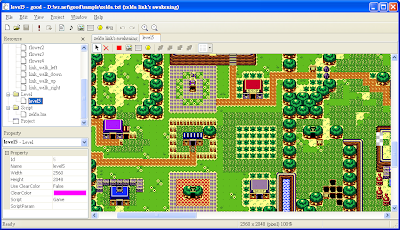先以編輯器編輯好遊戲資源。使用地圖TileSet編輯好地圖資源，並開一個新關卡資源加地圖加入。然後再加入幾個簡單的精靈資源，主要是林克的4個行走方向，還有幾朵不同顏色的花。接著就可以開始用Lua來實作Gameplay。

+ + +

+ + +

`Game.OnCreate = function(param) local idLvl = param._id scrolling = false Good.SetPos(idLvl, 320, 1280) KillAndDynaGenObj(idLvl)end`
Game初始化的時候，我們先把關卡的位置捲到小屋前林克所在的小地圖位置，同時初始化用來控制是否作地圖捲動的全域變數scrolling。接著再呼叫函式KillAndDynaGenObj來動態產生小花物件。

KillAndDynaGenObj函式首先會把除了林克以外的所有精靈物件刪除。接著檢查目前地圖可見範圍內的每一塊Tile，假如是小花Tile的話，則在那個地置動態的產生一個小花物件出來(全部共有4種不同顏色的小花)。最後一步則是再把林克物件加入關卡裡，這個動作是重新調整物件的次序，確保林克會畫在其它物件上面。
`function KillAndDynaGenObj(idLvl) local nc = Good.GetChildCount(idLvl) for i = nc,0,-1 do   local idChild = Good.GetChild(idLvl, i)   if (Good.TYPES_OBJ == Good.GetType(idChild) and 14 ~= idChild) then     Good.KillObj(idChild)   end end local x,y = Good.GetPos(idLvl) for i = 0,9 do   for j = 0,7 do     local lx,ly = x + 16 * i, y + 16 * j     local tile = Resource.GetTileByPos(1, lx, ly)     ...(略)   end end Good.AddChild(idLvl, 14)end`
OnStep則檢查scrolling全域變數是否設立來執行捲動，捲動的方向則檢查另一全域變數dir。捲動的時候依據水平或垂直方向決定速度，再配合offset全域變數來控制捲動量。當結束捲動時，再執行一次KillAndDynaGenObj函式重新產生小花物件。
`Game.OnStep = function(param) local idLvl = param._id if (not scrolling) then   return end local x,y = Good.GetPos(idLvl) local spd1, spd2 = 5, 4 if (Input.KEYS_LEFT == dir) then   x = x - spd1   offset = offset + spd1 elseif ...   ....(略) end Good.SetPos(idLvl, x, y) if (Input.KEYS_LEFT == dir or Input.KEYS_RIGHT == dir) then   if (160 <= offset) then     scrolling = false   end elseif (Input.KEYS_DOWN == dir or Input.KEYS_UP == dir) then   if (128 <= offset) then     scrolling = false   end  end  if (not scrolling) then    KillAndDynaGenObj(idLvl)  endend`

+ + +

`Link.OnStep = function(param) local id = param._id local x,y = Good.GetPos(id) if (scrolling) then   local spd1, spd2 = 0.5, 0.5   if (Input.KEYS_LEFT == dir) then     x = x - spd1   elseif ...     ....(略)   end   Good.SetPos(id, x, y)   return endend`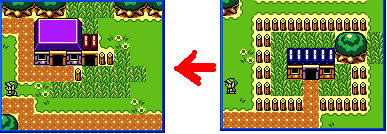`Link.OnStep = function(param)  ....(略)  local ox,oy = x,y  local spr  local spd = 0.8  if (Input.IsKeyDown(Input.KEYS_LEFT)) then    x = x - spd    spr = 10  elseif (Input.IsKeyDown(Input.KEYS_RIGHT)) then    x = x + spd    spr = 13  end  if (Input.IsKeyDown(Input.KEYS_DOWN)) then    y = y + spd    spr = 11  elseif (Input.IsKeyDown(Input.KEYS_UP)) then    y = y - spd    spr = 12  end  if (ox == x and oy == y) then    return  end  Good.SetSpriteId(id, spr)end`

`Link.OnStep = function(param)  ....(略)  local cx,cy = x + 8, y + 10  if (Input.IsKeyDown(Input.KEYS_LEFT)) then    cx = x;  elseif (Input.IsKeyDown(Input.KEYS_RIGHT)) then    cx = x + 16  end  if (Input.IsKeyDown(Input.KEYS_DOWN)) then    cy = y + 16  end  if (not IsMoveable(cx, cy)) then    return  endend`

`MoveableTile = {121,122,....(略)}function IsMoveable(x, y)  local tile = Resource.GetTileByPos(1, x, y)  for i,v in ipairs(MoveableTile) do    if (tile == v) then      return true    end  end  return falseend`
IsMoveable原理很簡單，根據指定位置取出地圖Tile，並且和MoveableTile裡所列出的所有可走的Tile作比較作是否可走判定。
`Link.OnStep = function(param)  ....(略)  local idLvl = Good.GetParent(id)  local px,py = Good.GetPos(idLvl)  dir = -1  if (px > x) then    dir = Input.KEYS_LEFT    x = x - 0.5  elseif (px + 160 < x + 16) then    dir = Input.KEYS_RIGHT    x = x + 0.5  end  if (py > y) then    dir = Input.KEYS_UP    y = y - 0.5  elseif (py + 128 < y + 16) then    dir = Input.KEYS_DOWN    y = y + 0.5  end    if (-1 ~= dir) then    offset = 0    scrolling = true  end  Good.SetPos(id, x, y)end`

....(完)

## 2009年5月1日 星期五

### 分解字串成Token

`string s("this is a string");vector<string> v;v.assign(  istream_iterator<string>(stringstream(s)),  istream_iterator<string>());`

getline可以提供這基本的需求。
`stringstream ss(s);string token;for (;;){  getline(ss, token, ',');  if (ss.fail())    break;  cout << token << endl;}`

+ + +

`stringstream ss;copy(  v.begin(), v.end(),  ostream_iterator<string>(ss, " "));string s(ss.str());`
stringstream的str方法也會產生一份新的string，所以當字串很大時效能也會受到影響。